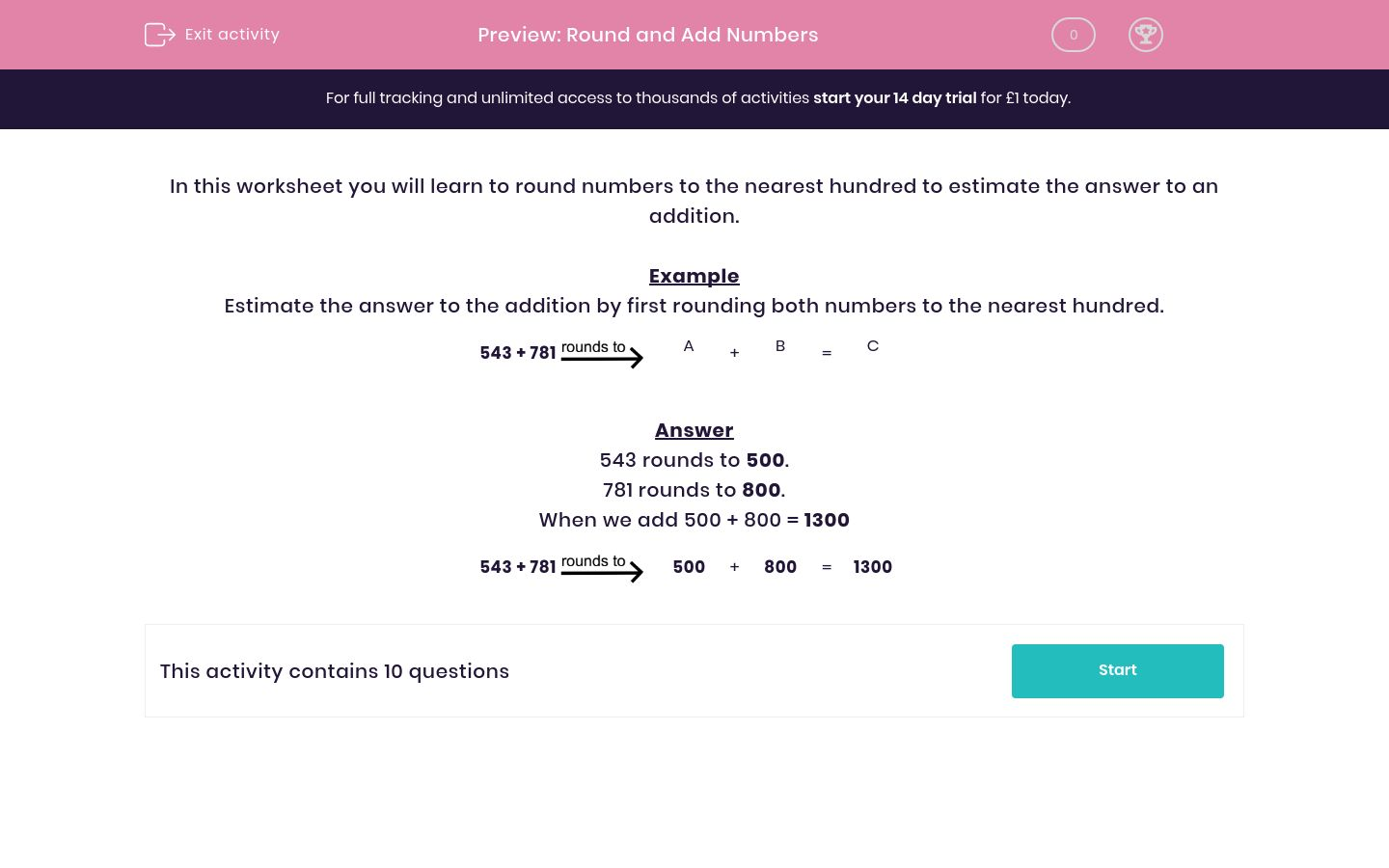### Affordable pricing from £10/month

In this worksheet, students learn to round numbers to the nearest hundred in order to estimate the answer to an addition.Key stage:  KS 2

Curriculum topic:   Maths and Numerical Reasoning

Curriculum subtopic:   Place Value

Difficulty level:### QUESTION 1 of 10

In this worksheet you will learn to round numbers to the nearest hundred to estimate the answer to an addition.

Example

Estimate the answer to the addition by first rounding both numbers to the nearest hundred.

543 + 781A

+

 B

=

 C

543 rounds to 500.

781 rounds to 800.

When we add 500 + 800 = 1300

543 + 781500

+

 800

=

 1300

Estimate the answer by rounding the numbers to the nearest 100 before adding to find C.

304 + 893A
+
 B
=
 C

## Column B

A
1200
B
300
C
900

Estimate the answer by rounding the numbers to the nearest 100 before adding to find C.

174 + 775A
+
 B
=
 C

## Column B

A
800
B
200
C
1000

Estimate the answer by rounding the numbers to the nearest 100 before adding to find C.

304 + 803A
+
 B
=
 C

## Column B

A
1100
B
800
C
300

Estimate the answer by rounding the numbers to the nearest 100 before adding to find C.

914 + 293A
+
 B
=
 C

## Column B

A
900
B
1200
C
300

Estimate the answer by rounding the numbers to the nearest 100 before adding to find C.

978 + 433A
+
 B
=
 C

## Column B

A
1400
B
1000
C
400

Estimate the answer by rounding the numbers to the nearest 100 before adding to find C.

192 + 723A
+
 B
=
 C

## Column B

A
900
B
200
C
700

Estimate the answer by rounding the numbers to the nearest 100 before adding to find C.

305 + 603A
+
 B
=
 C

## Column B

A
300
B
600
C
900

Estimate the answer by rounding the numbers to the nearest 100 before adding to find C.

944 + 136A
+
 B
=
 C

## Column B

A
900
B
1000
C
100

Estimate the answer by rounding the numbers to the nearest 100 before adding to find C.

834 + 676A
+
 B
=
 C

## Column B

A
800
B
1500
C
700

Estimate the answer by rounding the numbers to the nearest 100 before adding to find C.

499 + 150A
+
 B
=
 C

## Column B

A
200
B
500
C
700
• Question 1

Estimate the answer by rounding the numbers to the nearest 100 before adding to find C.

304 + 893A
+
 B
=
 C

## Column B

A
300
B
900
C
1200
• Question 2

Estimate the answer by rounding the numbers to the nearest 100 before adding to find C.

174 + 775A
+
 B
=
 C

## Column B

A
200
B
800
C
1000
• Question 3

Estimate the answer by rounding the numbers to the nearest 100 before adding to find C.

304 + 803A
+
 B
=
 C

## Column B

A
300
B
800
C
1100
• Question 4

Estimate the answer by rounding the numbers to the nearest 100 before adding to find C.

914 + 293A
+
 B
=
 C

## Column B

A
900
B
300
C
1200
• Question 5

Estimate the answer by rounding the numbers to the nearest 100 before adding to find C.

978 + 433A
+
 B
=
 C

## Column B

A
1000
B
400
C
1400
• Question 6

Estimate the answer by rounding the numbers to the nearest 100 before adding to find C.

192 + 723A
+
 B
=
 C

## Column B

A
200
B
700
C
900
• Question 7

Estimate the answer by rounding the numbers to the nearest 100 before adding to find C.

305 + 603A
+
 B
=
 C

## Column B

A
300
B
600
C
900
• Question 8

Estimate the answer by rounding the numbers to the nearest 100 before adding to find C.

944 + 136A
+
 B
=
 C

## Column B

A
900
B
100
C
1000
• Question 9

Estimate the answer by rounding the numbers to the nearest 100 before adding to find C.

834 + 676A
+
 B
=
 C

## Column B

A
800
B
700
C
1500
• Question 10

Estimate the answer by rounding the numbers to the nearest 100 before adding to find C.

499 + 150A
+
 B
=
 C

## Column B

A
500
B
200
C
700
---- OR ----

Sign up for a £1 trial so you can track and measure your child's progress on this activity.

### What is EdPlace?

We're your National Curriculum aligned online education content provider helping each child succeed in English, maths and science from year 1 to GCSE. With an EdPlace account you’ll be able to track and measure progress, helping each child achieve their best. We build confidence and attainment by personalising each child’s learning at a level that suits them.

Get started